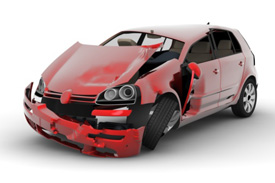Skip to content

# How to Measure The Force In A Car AccidentIf you have ever read an accident reconstruction report?

The conclusion always comes down to the same thing.

“The delta V was not high enough to cause any injury.”

The major problem with that statement is that delta V is not the important number.

What is important is the time it took for delta V or in physics terms, the acceleration.

Delta V is defined as the amount of change to the velocity/speed.

Acceleration is defined as the rate of speed change.over time.

Let’s see if you got this:

A plane going 550 MPH lands at Logan airport. What is the delta v?

If the plane lands in a typical fashion, wheels first, do you get hurt with such a huge delta V?

Answer: no, because the rate of acceleration (in this case negative acceleration) is very slow. It takes about 40 minutes to slow down.

If the rate of slowing down is sped up, like hitting a mountain will you get hurt?

Answer: yes because the rate of slowing down was increased rapidly from 40 minutes to less than a second.

## Calculating car accident force

acceleration (a) (avg)= delta v= change in velocity

delta t change in time

### Normal landing: we will call this event #1

a(avg)= 550 MPH = 806 feet per second = .335 feet per second
2400 seconds

### Crash Landing: we will call this event #2

a(avg)= 550 MPH = 806 feet per second = 4030 feet per second
.2 seconds

.2 seconds is how fast the average car crash is expected to be.

Your acceleration is much, much higher!

Now the next step is to figure out the force your events created:

Force= mass x acceleration

A 737 weighs approximately 175,000 lbs fully loaded

Event 1:
Force= 175000 x .335

F= 58,625

g’s= a avg = .335 = .01 g’s

gravity .32.2

### Event 2:

Force= 175,000 x 4030
Force = 705,250,000

Twelve thousand times the force in the crash.

g’s= a avg = 4030 = 125 g’s

gravity .32.2

## HUGE DIFFERENCES!

The bottom line is that crashes and the injuries sustained are all about the the time:

The typical auto crash happens in less then .2 seconds.

If the car does not break or crush the time is reduced, therefore creating much more force.

If the car does break or crush, the time is increased and the force is reduced.

This is the fallacy of no car damage no injury rhetoric.

In addition, the car bumpers of today are supposed to crush or break at 5 mph.

Most do not break until 10 mph.

When the bumper does not break, the bumper does an elastic rebound and magnifies the force.